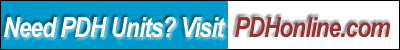Print this page

Errors and Confidence Intervals

David J. Lilja, Ph.D., P.E.

Course Overview

All measurements of real computer systems are subject to both random and systematic errors. These errors introduce uncertainty and imprecision into your measurements, which can make it difficult to interpret your results. In this course, you will learn how an appropriate model of these errors can be used to quantify the precision of your measurements using confidence intervals.

This course includes a multiple-choice quiz at the end, which is designed to enhance the understanding of the course materials.

Learning Objective

After completing this 3-hour course, you will be able to:

• Understand why errors are typically modeled using the Gaussian (normal) distribution;
• Understand the differences between the accuracy, precision, and resolution of your measuring tools;
• Calculate confidence intervals for the mean value of a collection of measurements;
• Calculate confidence intervals for proportions; and
• Estimate the number of measurements required to obtain a given level of precision in computing a sample mean.

The reading assignment for this course is Chapter 4 of Measuring Computer Performance: A Practitioner's Guide, David J. Lilja, Cambridge University Press, 2000.

If you don't have this book, you can purchase Chapter 1 in PDF format online at eBooks.com for a modest cost. The price for this course listed on this website does not include the cost of purchasing the chapter through eBooks.com. However, the price has been reduced to compensate for the cost of purchasing the chapter required. If you plan to take all 6 courses (E132 to E137) based on this book, you may consider to purchase a hard copy of the book or the entire book in PDF format online through eBooks.com.

Key Terms

• Accuracy
• Precision
• Resolution
• Random errors
• Systematic errors
• Gaussian error distribution
• Confidence interval
• Confidence level
• Significance level
• Central limit theorem

Study Notes

All measurements of real computer systems, such as those made using interval timers, for example, will include errors. These errors introduce some uncertainty into your measurements, which can make it difficult to interpret your final results. These errors are due to perturbations in the system being measured from time-sharing, interrupts, real-time processing, non-deterministic cache and memory replacement policies, and so forth. Additionally, the inherent accuracy, precision, and resolution limitations of your measuring tool will add errors to your final measured values.

You will learn in this course why the Gaussian probability distribution is typically used to model errors in most types of measurements that you are likely to make of computer systems. This distribution also is known as a normal distribution and is what we more casually refer to as the traditional bell curve.

If the distribution of errors in a set of measurements actually is Gaussian distributed, we can use the unique properties of this distribution to quantify the precision of the measurements. In particular, when we make a series of measurements, we compute the sample mean as our best estimate of the actual mean of the event being measured. We then compute a confidence interval for this sample mean. This confidence interval allows us to say how likely it is that the real mean is between the two end-points of the computed interval.

In this course, you will learn how to compute confidence intervals for both continuous values and for proportions. You also will learn how to use confidence intervals to estimate how many measurements you will have to make of a given system to obtain a desired level of precision in your estimate of the mean value.

Quiz

Once you finish studying the above course content, you need to take a quiz to obtain the PDH credits.

DISCLAIMER: The materials contained in the online course are not intended as a representation or warranty on the part of PDH Center or any other person/organization named herein. The materials are for general information only. They are not a substitute for competent professional advice. Application of this information to a specific project should be reviewed by a registered architect and/or professional engineer/surveyor. Anyone making use of the information set forth herein does so at their own risk and assumes any and all resulting liability arising therefrom.